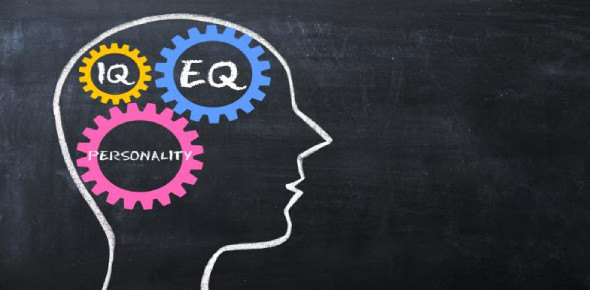# IQ Quiz For Dummies

Approved & Edited by ProProfs Editorial Team
The editorial team at ProProfs Quizzes consists of a select group of subject experts, trivia writers, and quiz masters who have authored over 10,000 quizzes taken by more than 100 million users. This team includes our in-house seasoned quiz moderators and subject matter experts. Our editorial experts, spread across the world, are rigorously trained using our comprehensive guidelines to ensure that you receive the highest quality quizzes.
| By Jmslipknot
J
Jmslipknot
Community Contributor
Quizzes Created: 1 | Total Attempts: 6,997
Questions: 6 | Attempts: 7,024SettingsHow smart are you? Multiple choice quiz.

• 1.

### Which planet can float?

• A.

Earth

• B.

Saturn

• C.

Mars

• D.

Neptune

• E.

Venus

B. Saturn
Explanation
Saturn is the correct answer because it is the only planet in our solar system that has a density less than that of water. This means that if there were a large enough body of water, Saturn would be able to float on it. The low density of Saturn is due to its composition, which includes large amounts of hydrogen and helium gases.

Rate this question:

• 2.

### What number comes next?                 1,2,5,14,41...

• A.

75

• B.

123

• C.

87

• D.

94

• E.

Micheal Jakson

B. 123
Explanation
The sequence is obtained by multiplying the previous number by 2 and subtracting 1. Starting with 1, we have 1*2 - 1 = 1, then 2*2 - 1 = 3, then 3*2 - 1 = 5, and so on. Therefore, the next number in the sequence would be 41*2 - 1 = 81 - 1 = 80.

Rate this question:

• 3.

### What weighs the most?

• A.

10 lbs of propane

• B.

10 lbs of feathers

• C.

A+B

• D.

None of the above

• E.

Not enough info

C. A+B
Explanation
The answer "A+B" is correct because it implies that the combined weight of both A and B is the heaviest. Without any further information or context about the objects A and B, we cannot determine their individual weights or compare them to other options like 10 lbs of propane or 10 lbs of feathers. Therefore, the correct answer is A+B.

Rate this question:

• 4.

### What's the sexiest color?

• A.

Purple

• B.

Red

• C.

Blue

• D.

Green

• E.

Umm... you spelled colour wrong

A. Purple
Explanation
The question is subjective and does not have a definitive answer. However, purple is often associated with luxury, royalty, and sensuality, which can be considered sexy by some individuals.

Rate this question:

• 5.

### How many months have 30 days

• A.

11

• B.

All of them

• C.

6

• D.

8 (only on leap year)

• E.

3

A. 11
Explanation
There are 11 months that have 30 days. This is because all months except February have either 30 or 31 days. So, the correct answer is 11.

Rate this question:

• 6.

### What animal uses the most tools?

• A.

Chimps

• B.

Dolphins

• C.

Sharks

• D.

Cats

• E.

None of the above

E. None of the above
Explanation
None of the above options correctly answer the question. While chimpanzees are known to use tools in the wild, they are not the animal that uses the most tools. Dolphins, sharks, and cats do not generally use tools at all. Therefore, the correct answer is none of the above.

Rate this question:

Related TopicsBack to top